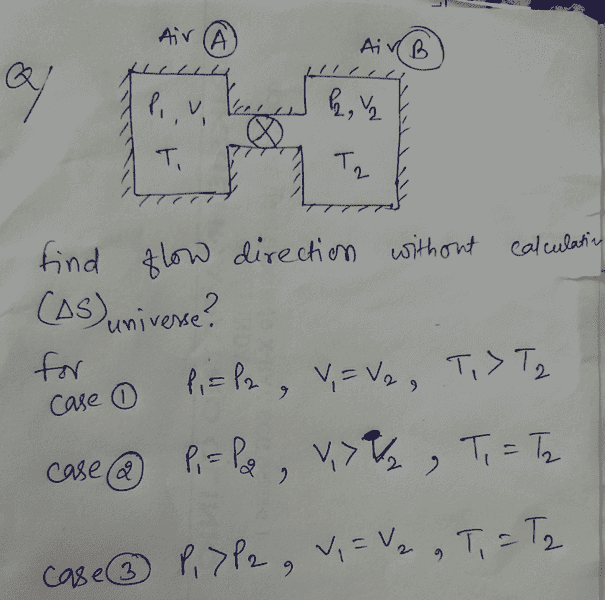# What will be the flow direction?I guess there would be no flow in case 2; case 3 seems easy to me applying fluid mechanics principle; flow will be 1 to 2. But I doubt case 1; it is not in thermal equilibrium ??? Pls help

Chestermiller
Mentor
Can heat flow between the two chambers, or are the insulated from one another?

BvU
Homework Helper
You may assume the system will tend to go to equilibrium. What does that mean for T1 and T2 ? If they don't stay the same, then what does the change in T1 mean for P1 ?

Yeah, heat flow is allowed between chambers;
What you are seeing is just a valve between two chambers.

You may assume the system will tend to go to equilibrium. What does that mean for T1 and T2 ? If they don't stay the same, then what does the change in T1 mean for P1 ?

It doesn't seem practical to me also. So pls answer one that you want to acknowledge.

BvU
Homework Helper
It doesn't seem practical to me also. So pls answer one that you want to acknowledge.
Could you replhrase ? I don't understand what you are trying to tell me. Basically I don't answer: I help answering by asking questions. About case 1.

Could you replhrase ? I don't understand what you are trying to tell me. Basically I don't answer: I help answering by asking questions. About case 1.

Pressure is equal before; but temperature T1 is more----> They would try to attain the thermal equilibrium. When valve is being opened; I don't know what would happen; simply because I am not able to think; because I don't have numerical values how can I compare the density (say). I could say if there is density variation due to temperature difference simply flow will be from higher density to lower density side.

BvU
Homework Helper
You have the ideal gas law for guidance.

I could say if there is density variation due to temperature difference simply flow will be from higher density to lower density side.
Is it right approach?

BvU
Homework Helper
Check out ##p_1V_1 = n_1RT_1## in combination with ##p_2V_2 = n_2RT_2## in combination with ##p_1 = p_2##, ##V_1=V_2## and ##T_1 > T_2##. What about ##n_1'## and ##n_2'## after equilibrium is established ?

•gracy
Why can't we apply simple fundamental principle that heat flows from higher temperature to lower temperature?

Last edited:
BvU
Homework Helper
We do apply that. But to answer the question what that means for the material flow we need a follow-up step.

Chestermiller
Mentor
CASE 1: According to the ideal gas law, how many moles of gas are present in container 1 before the valve is open? How many moles of gas are present in container 2 before the valve is open? Does the total number of moles of gas in the two containers change after the valve is open? In the final equilibrium state, are the temperatures in the two containers (a) the same or (b) different? (If they are the same, then call that T). Does the overall system do any work on the surroundings? Does the overall system exchange any heat with the surroundings? From the first law of thermodynamics, what does that tell you about the change in internal energy of the overall system? From this, what is the final temperature? What is the final pressure? What are the final number of moles of gas present in container 1? How does that compare with the initial number of moles of gas present in container 1?

•BvU
Chestermiller
Mentor
Better yet, if the final temperatures, pressures, and volumes are equal, what does that tell you about the final ratio of the number of moles of gas in each container?

That means in all the cases; simply we have to find the ratio of moles of two chamber. Flow direction will be from higher to lower number of moles.

Chestermiller
Mentor
That means in all the cases; simply we have to find the ratio of moles of two chamber. Flow direction will be from higher to lower number of moles.
Not in case 2. In case 2, there will be no flow even though there are more moles in chamber 2.

Not in case 2. In case 2, there will be no flow even though there are more moles in chamber 2.
I think more moles will in chamber 1 in case 2.

Chestermiller
Mentor
I think more moles will in chamber 1 in case 2.
Sorry. I got it backwards.